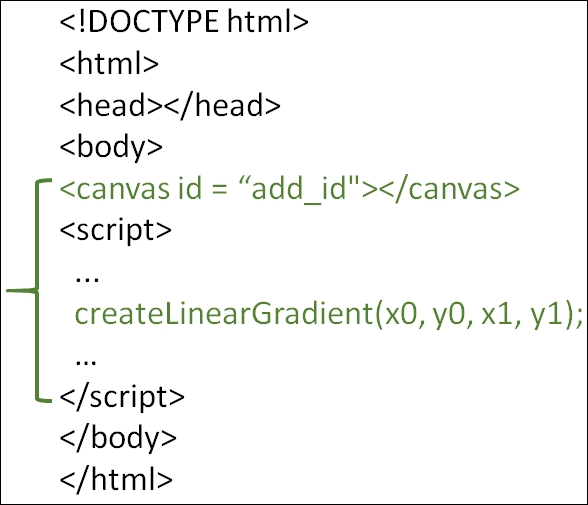# How to draw a linear gradient in HTML5?

HTMLWeb DevelopmentFront End Technology

This method returns a CanvasGradient object that represents a linear gradient that paints along the line given by the coordinates represented by the arguments. The four arguments represent the starting point (x1,y1) and end point (x2,y2) of the gradient.

createLinearGradient(x0, y0, x1, y1)

Here are the parameter values of the createLinearGradient() method −

S.No
Parameter & Description
1
x0
x-coordinate- Starting point of the gradient
2
y0
y- coordinate - Starting point of the gradient
3
x1
x-coordinate - Ending point of the gradient
4
y1
y- coordinate - Ending point of the gradient## Example

You can try to run the following code to learn how to draw a linear gradient in HTML5 −

<!DOCTYPE html>
<html>

<body>
<canvas id="newCanvas" width="450" height="250" style="border:1px
solid #d3d3d3;"></canvas>
<script>
var c = document.getElementById("newCanvas");
var ctxt = c.getContext("2d");
var linegrd = ctxt.createLinearGradient(0, 0, 170, 0);
</html>